这又是怎么做出来的呢，似乎让人有点摸不着头脑。我们来先来看看他的代码（注意红色部分的代码）

<table width="420" border="0" cellspacing="2" cellpadding="1" height="10">
<tr>
<td bgcolor="#000000">
<table width="100%" border="0" cellspacing="0" cellpadding="0" height="100%">
<tr>
<td bgcolor="#FFFFFF"> </td>
</tr>
</table>
</td>
<td bgcolor="#000000">
<table width="100%" border="0" cellspacing="0" cellpadding="0" height="100%">
<tr>
<td bgcolor="#FFFFFF"> </td>
</tr>
</table>
</td>
<td bgcolor="#000000">
<table width="100%" border="0" cellspacing="0" cellpadding="0" height="100%">
<tr>
<td bgcolor="#FFFFFF"> </td>
</tr>
</table>
</td>
<td bgcolor="#000000">
<table width="100%" border="0" cellspacing="0" cellpadding="0" height="100%">
<tr>
<td bgcolor="#FFFFFF"> </td>
</tr>
</table>
</td>
<td bgcolor="#000000">
<table width="100%" border="0" cellspacing="0" cellpadding="0" height="100%">
<tr>
<td bgcolor="#FFFFFF"> </td>
</tr>
</table>
</td>
<td bgcolor="#000000">
<table width="100%" border="0" cellspacing="0" cellpadding="0" height="100%">
<tr>
<td bgcolor="#FFFFFF"> </td>
</tr>
</table>
</td>
</tr>
</table>

<table width="100%" border="0" cellspacing="0" cellpadding="0" height="100%">
<tr>
<td> </td>
</tr>
</table>

详细的操作步骤如下：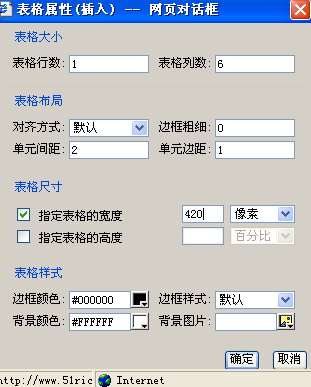第二步：单击“显示隐藏指导方针”按钮，将该表格每个单元格的背景色设置为黑色（注意：是单元格而不是整个表格，方法是把光标分别放在各个单元格里，单击“单元格属性”按钮，将背景色设为黑色），设置完后表格显示如下：第三步：分别在每个单元格中再插入一个1行1列的表格（边框粗细、单元格间距和单元格边距都设为0，表格背景色设为白色）即可。

需要注意的就是上面代码中加了红色的属性一个也不能少！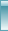建站技巧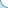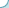站内搜索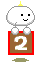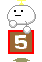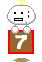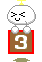公司地址：深圳市福田区福民路12号知本大厦706   邮编：518000         客服热线：0755-61192828 传真：0755-82915763  客服的服务质量投诉::service@51rich.net(如果您觉得哪位客服的服务不好或者服务得超级好,都可以发EM通知我们,并写上原因,谢谢您的监督) 网站管理